## Описание презентации Physics of Semiconductor Devices Lecture 1. Introduction по слайдам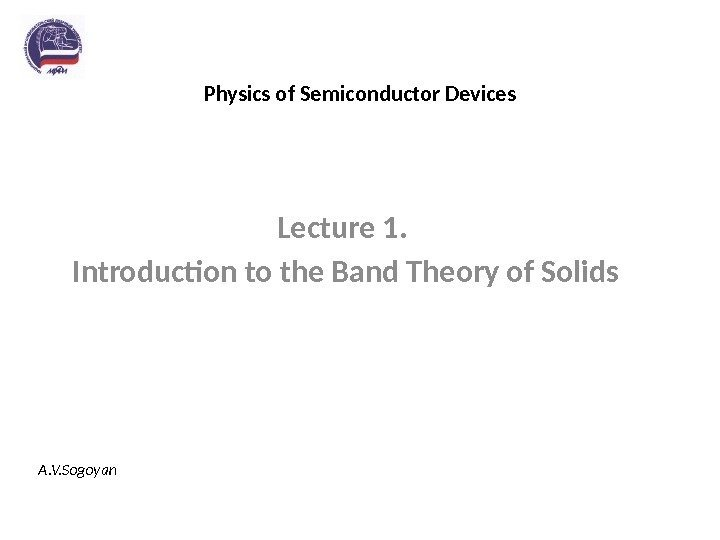Physics of Semiconductor Devices Lecture 1. Introduction to the Band Theory of Solids A. V. Sogoyan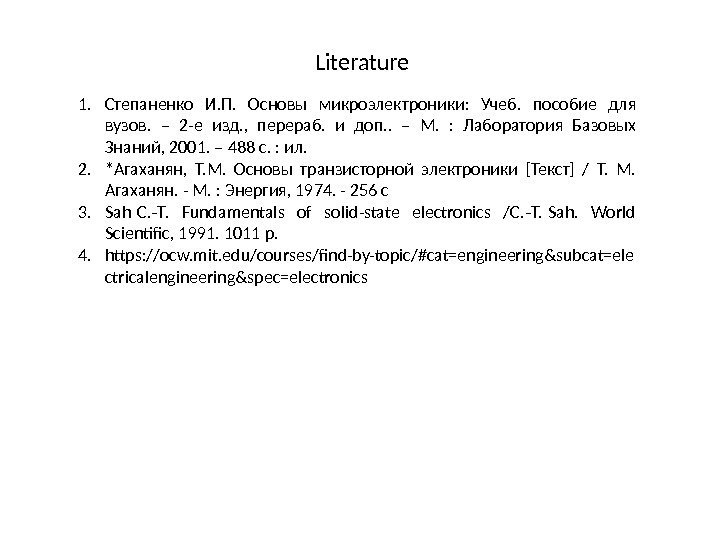Literature 1. Степаненко И. П. Основы микроэлектроники: Учеб. пособие для вузов. – 2 -е изд. , перераб. и доп. . – М. : Лаборатория Базовых Знаний, 2001. – 488 с. : ил. 2. *Агаханян, Т. М. Основы транзисторной электроники [Текст] / Т. М. Агаханян. — М. : Энергия, 1974. — 256 с 3. Sah C. T. Fundamentals of solid-state electronics /C. T. Sah. World ‑ ‑ Scientific, 1991. 1011 p. 4. https: //ocw. mit. edu/courses/find-by-topic/#cat=engineering&subcat=ele ctricalengineering&spec=electronics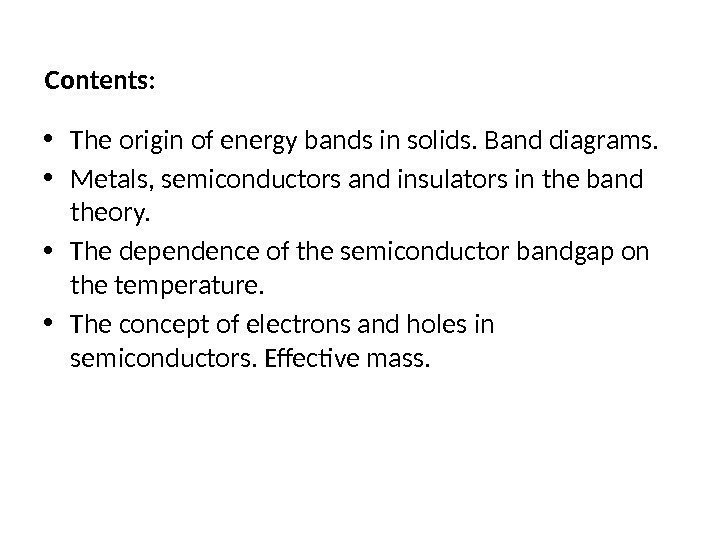Contents: • The origin of energy bands in solids. Band diagrams. • Metals, semiconductors and insulators in the band theory. • The dependence of the semiconductor bandgap on the temperature. • The concept of electrons and holes in semiconductors. Effective mass.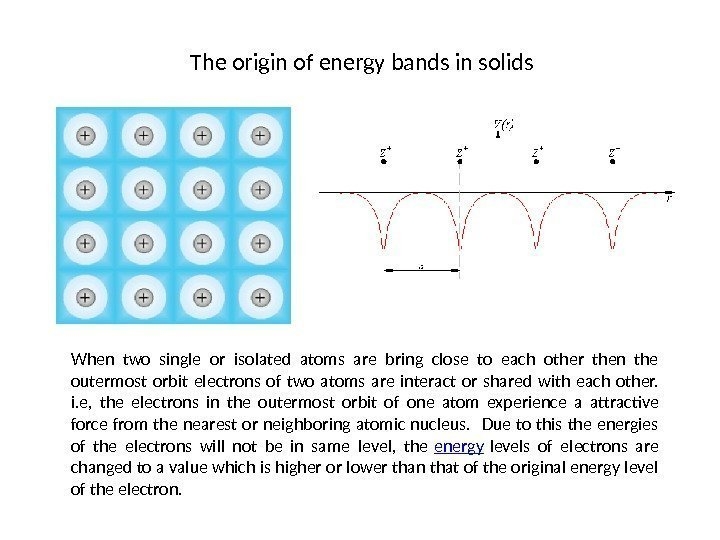The origin of energy bands in solids When two single or isolated atoms are bring close to each other then the outermost orbit electrons of two atoms are interact or shared with each other. i. e, the electrons in the outermost orbit of one atom experience a attractive force from the nearest or neighboring atomic nucleus. Due to this the energies of the electrons will not be in same level, the energy levels of electrons are changed to a value which is higher or lower than that of the original energy level of the electron.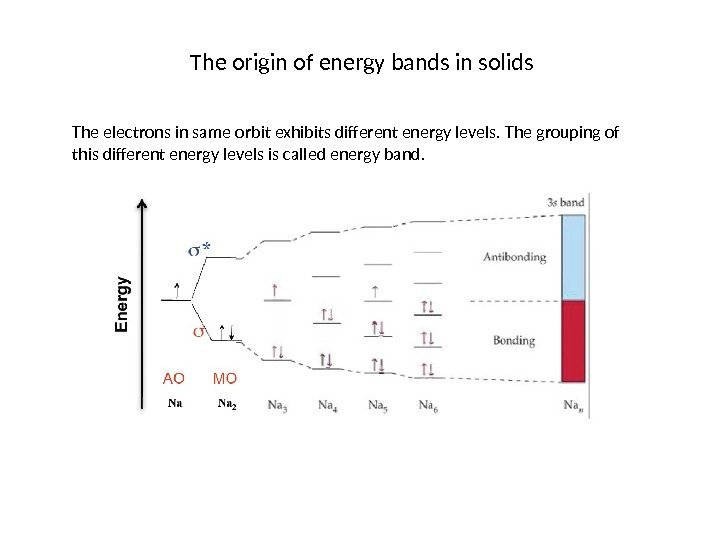The origin of energy bands in solids The electrons in same orbit exhibits different energy levels. The grouping of this different energy levels is called energy band.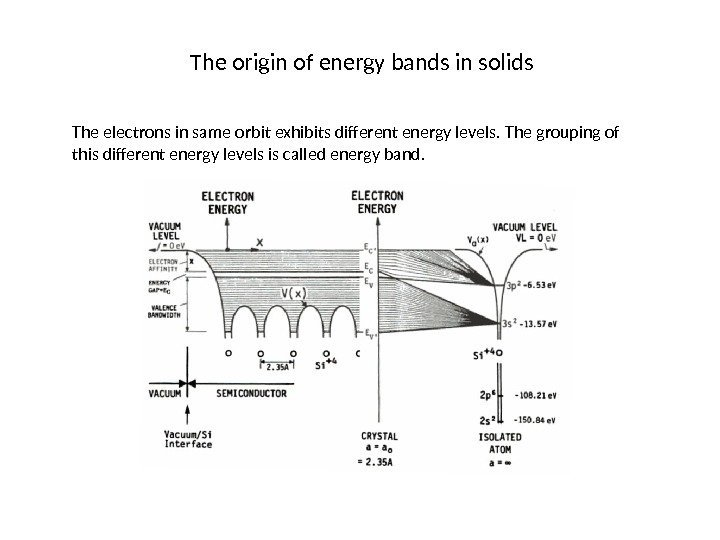The origin of energy bands in solids The electrons in same orbit exhibits different energy levels. The grouping of this different energy levels is called energy band.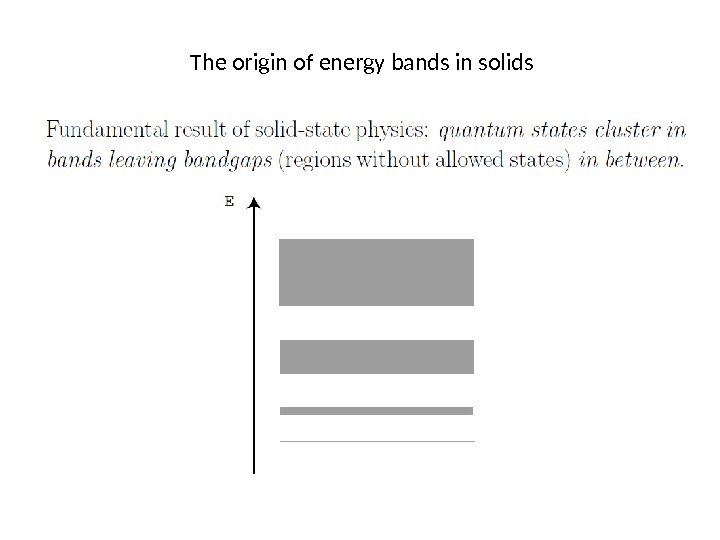The origin of energy bands in solids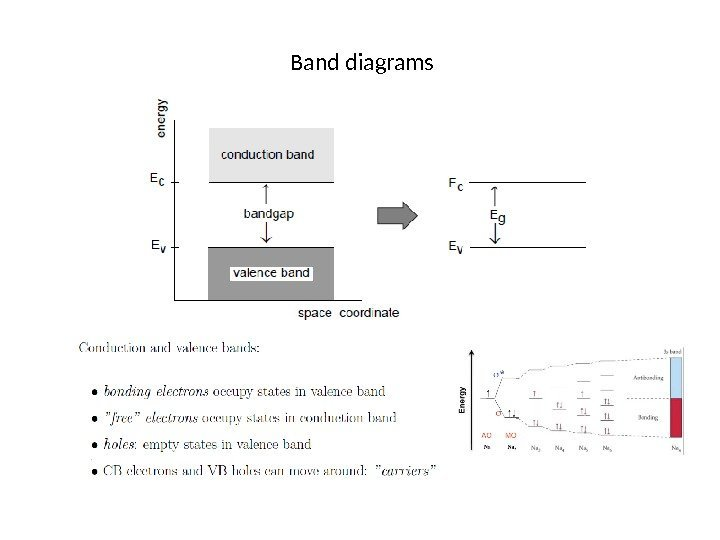Band diagrams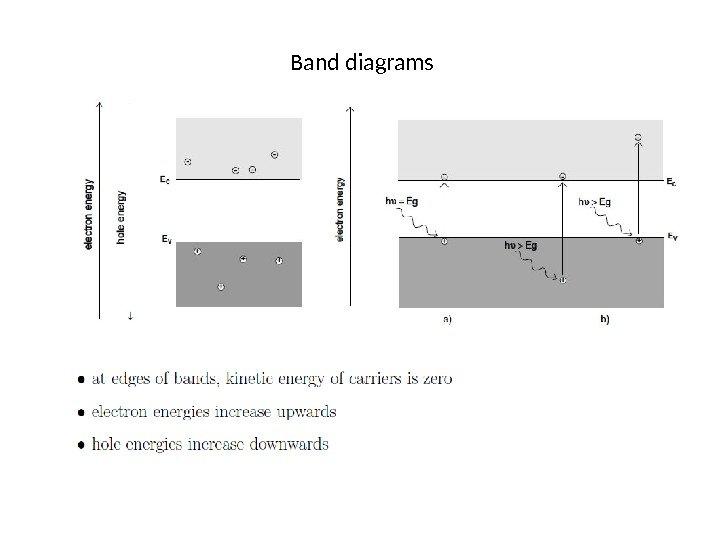Band diagrams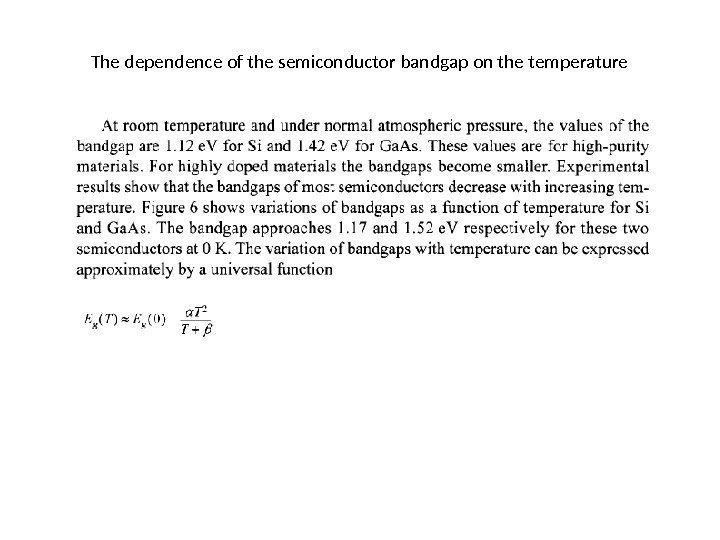The dependence of the semiconductor bandgap on the temperature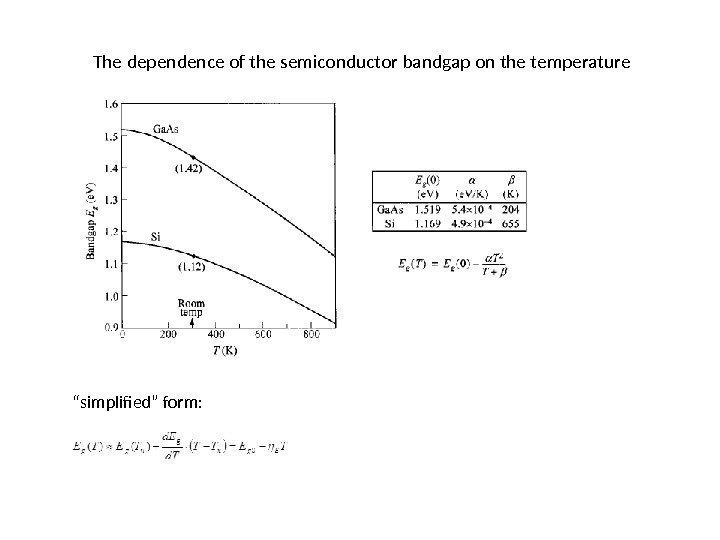The dependence of the semiconductor bandgap on the temperature “ simplified” form: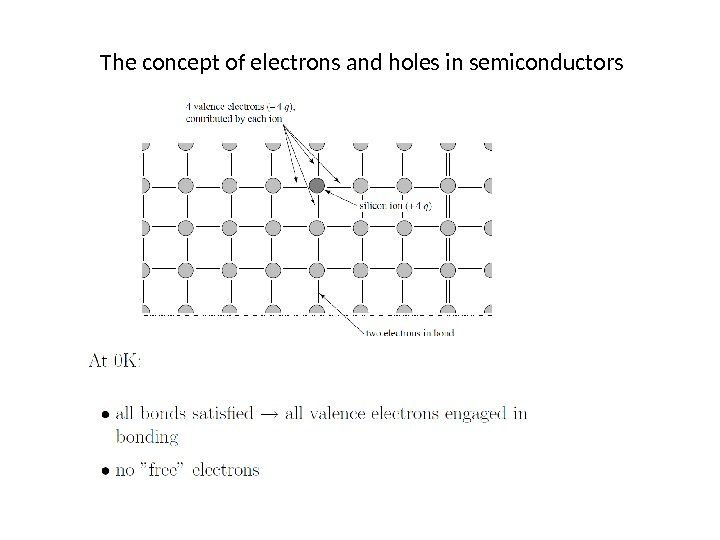The concept of electrons and holes in semiconductors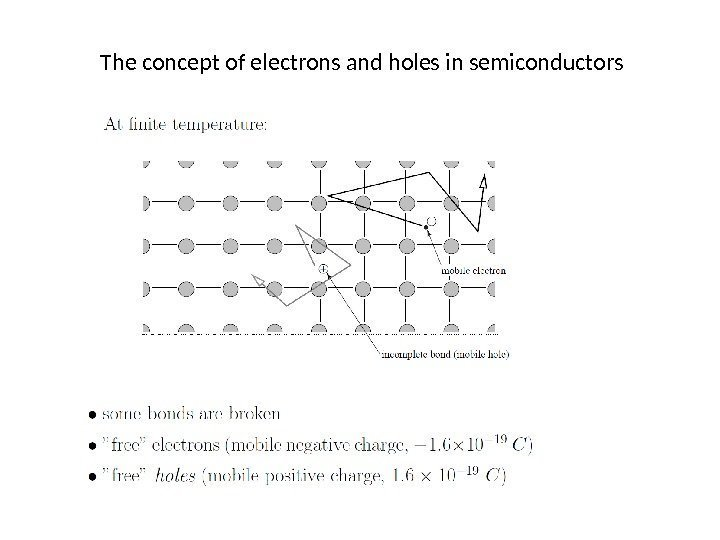The concept of electrons and holes in semiconductors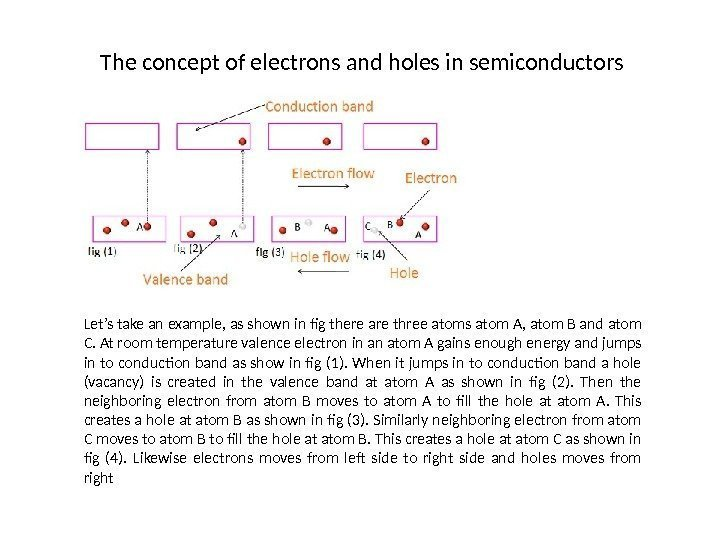The concept of electrons and holes in semiconductors Let’s take an example, as shown in fig there are three atoms atom A, atom B and atom C. At room temperature valence electron in an atom A gains enough energy and jumps in to conduction band as show in fig (1). When it jumps in to conduction band a hole (vacancy) is created in the valence band at atom A as shown in fig (2). Then the neighboring electron from atom B moves to atom A to fill the hole at atom A. This creates a hole at atom B as shown in fig (3). Similarly neighboring electron from atom C moves to atom B to fill the hole at atom B. This creates a hole at atom C as shown in fig (4). Likewise electrons moves from left side to right side and holes moves from right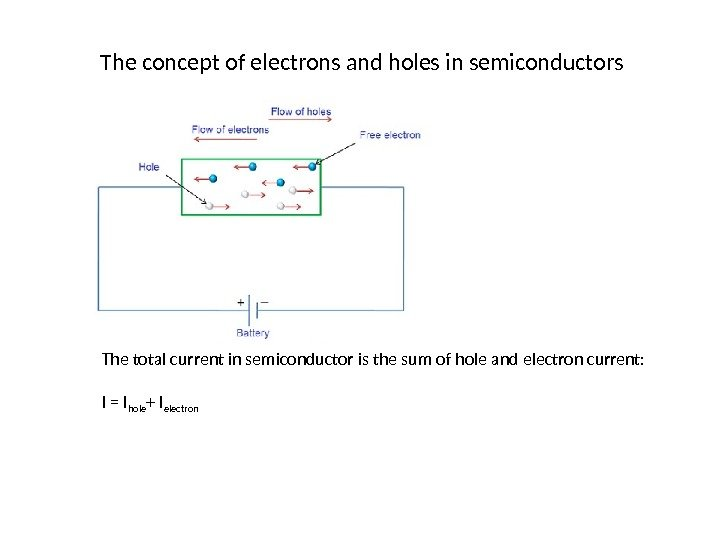The concept of electrons and holes in semiconductors The total current in semiconductor is the sum of hole and electron current: I = I hole + I electron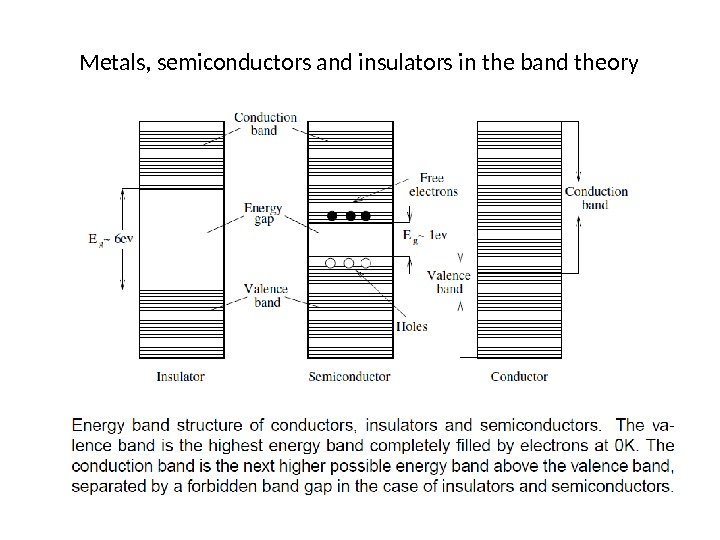Metals, semiconductors and insulators in the band theory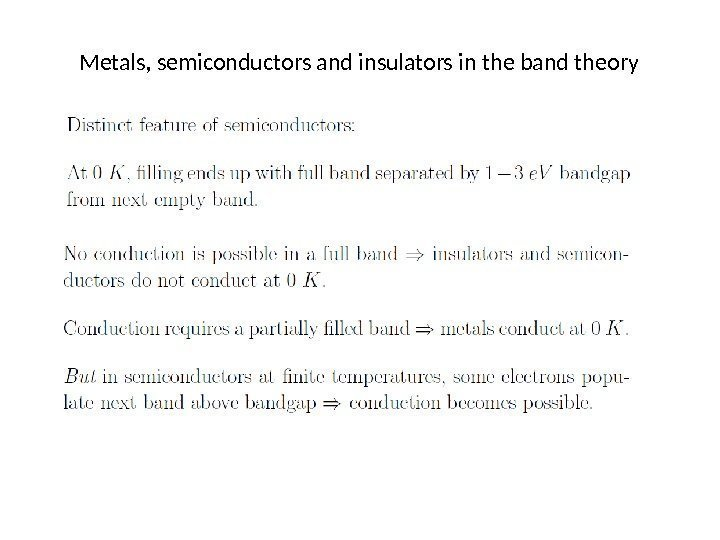Metals, semiconductors and insulators in the band theory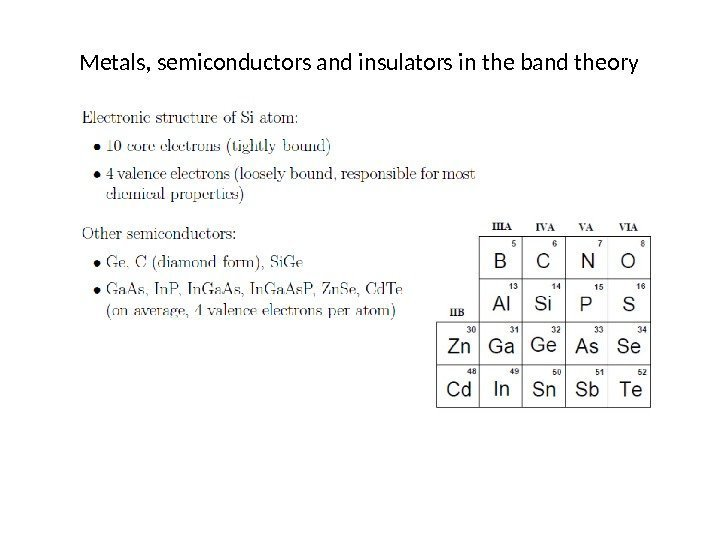Metals, semiconductors and insulators in the band theory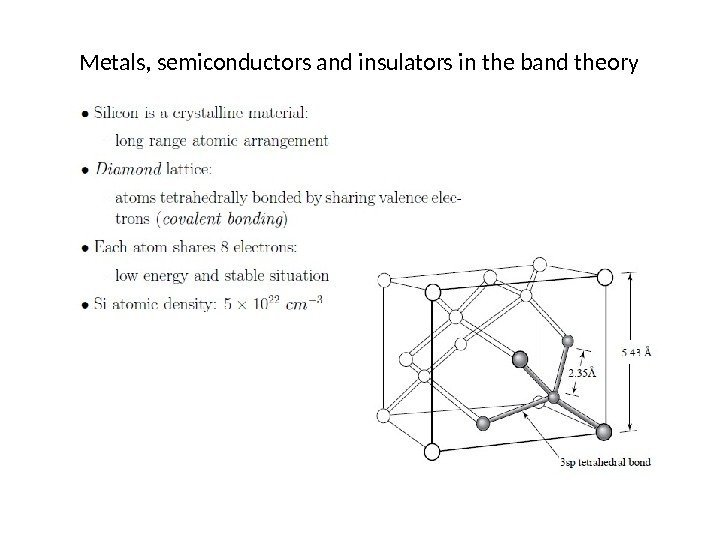Metals, semiconductors and insulators in the band theory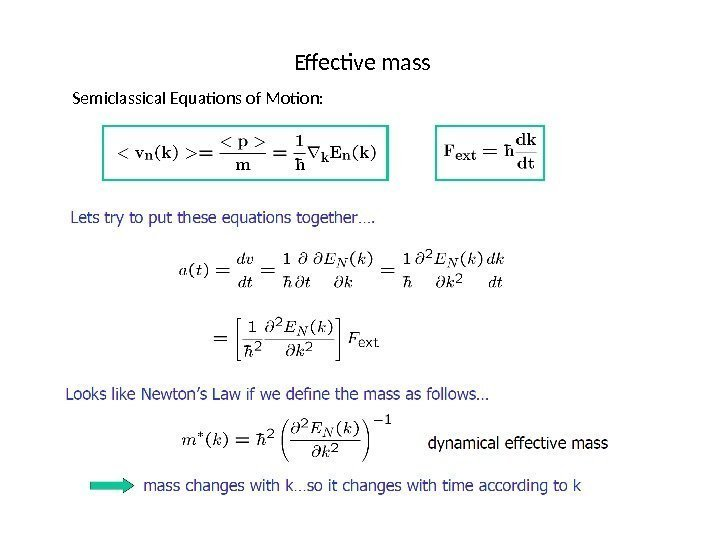Effective mass Semiclassical Equations of Motion: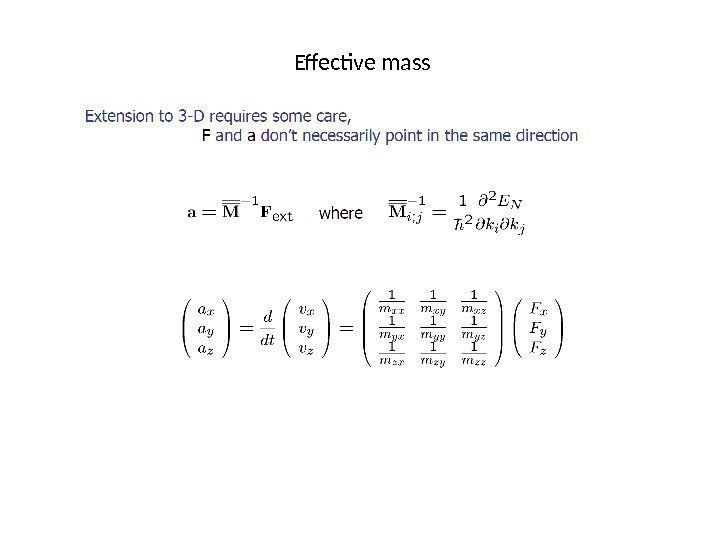Effective mass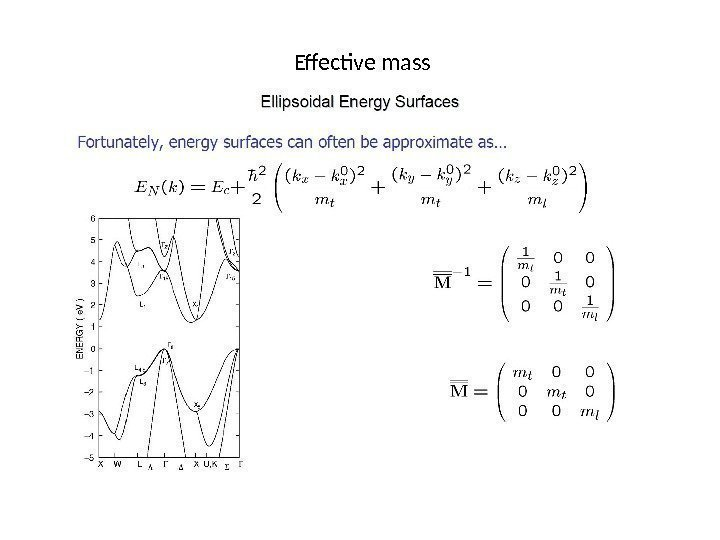Effective mass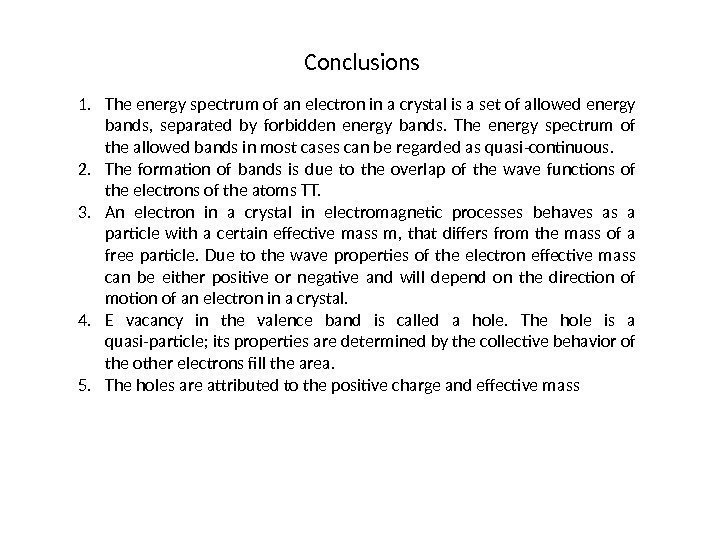Conclusions 1. The energy spectrum of an electron in a crystal is a set of allowed energy bands, separated by forbidden energy bands. The energy spectrum of the allowed bands in most cases can be regarded as quasi-continuous. 2. The formation of bands is due to the overlap of the wave functions of the electrons of the atoms TT. 3. An electron in a crystal in electromagnetic processes behaves as a particle with a certain effective mass m, that differs from the mass of a free particle. Due to the wave properties of the electron effective mass can be either positive or negative and will depend on the direction of motion of an electron in a crystal. 4. E vacancy in the valence band is called a hole. The hole is a quasi-particle; its properties are determined by the collective behavior of the other electrons fill the area. 5. The holes are attributed to the positive charge and effective mass